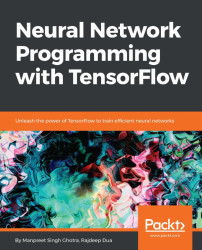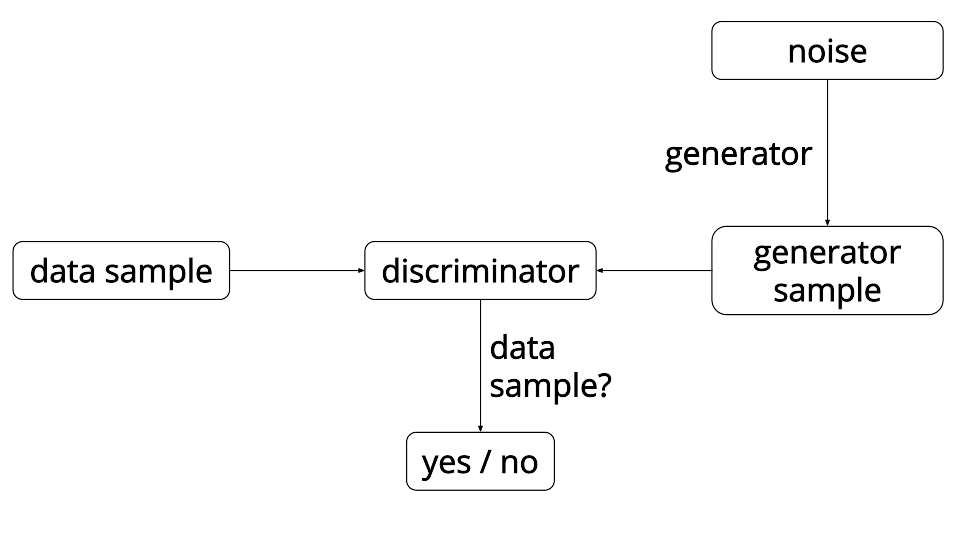•#### Neural Network Programming with Tensorflow#### Overview of this book

If you're aware of the buzz surrounding the terms such as "machine learning," "artificial intelligence," or "deep learning," you might know what neural networks are. Ever wondered how they help in solving complex computational problem efficiently, or how to train efficient neural networks? This book will teach you just that. You will start by getting a quick overview of the popular TensorFlow library and how it is used to train different neural networks. You will get a thorough understanding of the fundamentals and basic math for neural networks and why TensorFlow is a popular choice Then, you will proceed to implement a simple feed forward neural network. Next you will master optimization techniques and algorithms for neural networks using TensorFlow. Further, you will learn to implement some more complex types of neural networks such as convolutional neural networks, recurrent neural networks, and Deep Belief Networks. In the course of the book, you will be working on real-world datasets to get a hands-on understanding of neural network programming. You will also get to train generative models and will learn the applications of autoencoders. By the end of this book, you will have a fair understanding of how you can leverage the power of TensorFlow to train neural networks of varying complexities, without any hassle. While you are learning about various neural network implementations you will learn the underlying mathematics and linear algebra and how they map to the appropriate TensorFlow constructs.
Title PageCreditswww.PacktPub.comCustomer FeedbackPrefaceFree Chapter
Maths for Neural NetworksDeep Feedforward NetworksOptimization for Neural NetworksConvolutional Neural NetworksRecurrent Neural NetworksGenerative ModelsDeep Belief NetworkingAutoencodersResearch in Neural NetworksGetting started with TensorFlow## GANs

GANs were introduced by a group of researchers at the University of Montreal led by Ian Goodfellow. The core idea behind a GAN model is to have two competing neural network models. One network takes the noise as input and generates samples (hence known as generator). The second model (known as discriminator) gets samples from both the generator and the actual training data, and should be able to differentiate between the two sources. Generative and discriminative networks are playing a continuous game, where the generator model is learning to generate more realistic samples or examples, and the discriminator is learning to get better and better at differentiating generated data from the real data. The two networks are trained simultaneously, and the goal is that the competition will make the generated samples indistinguishable from the real data:The analogy used to describe GANs is that the generator is like a forger that is attempting to produce some forged material, and the discriminator...